# Electromagnetics - Online Test

Q1.
Two electrodes, whose cross-sectional view is shown in the figure below, are at the same potential. The maximum electric field will be at the point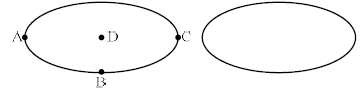Explaination / Solution: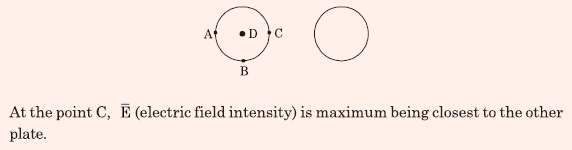Q2. Consider an electron, a neutron and a proton initially at rest and placed along a straight line such that the neutron is exactly at the center of the line joining the electron and proton. At t=0, the particles are released but are constrained to move along the same straight line. Which of these will collide first?
Explaination / Solution: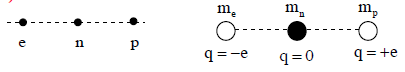Q3. A solid iron cylinder is placed in a region containing a uniform magnetic field such that the cylinder axis is parallel to the magnetic field direction. The magnetic field lines inside the cylinder will
Explaination / Solution:

Flux always chooses less reluctance path. So flux tried to flow inside the conductor and closer to the axis of the cylinder.

Q4. Divergence of the three-dimensional radial vector fieldis
Explaination / Solution:
No Explaination.

Q5. If C is code curve enclosing a surface S , then magnetic field intensity, the current densityand the electric flux density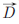are related by
Explaination / Solution: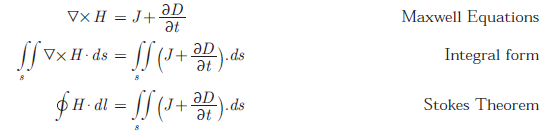Q6. It is given that X, X .... XM at M non-zero, orthogonal vectors. The dimension of the vector space spanned by the 2M vectors X, X, ....XM, - X, - X, ..... - Xis
Explaination / Solution:

For two orthogonal vectors, we require two dimensions to define them and similarly for three orthogonal vector we require three dimensions to define them. 2M vectors are basically M orthogonal vector and we require M dimensions to define them.

Q7. The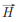field (in A/m) of a plane wave propagating in free space is given byThe time average power flow density in Watts is
Explaination / Solution: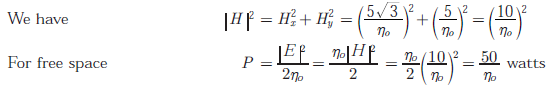Q8. For static electric and magnetic fields in an inhomogeneous source-free medium, which of the following represents the correct form of Maxwell’s equations ?
Explaination / Solution: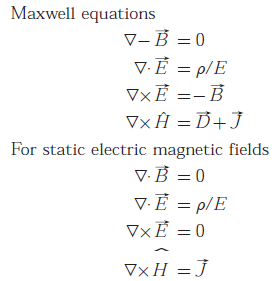Q9. The value of the integral of the function g(x,y) = 4x3 + 10y4 along the straight line segment from the point (0,0) to the point (1,2) in the x - y plane is
Explaination / Solution: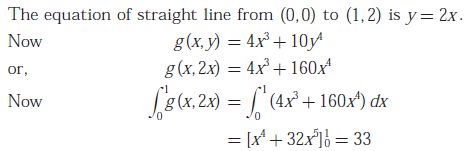Q10. A uniform plane wave in the free space is normally incident on an infinitely thick dielectric slab (dielectric constant ε = 9). The magnitude of the reflection coefficient is
Explaination / Solution: